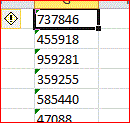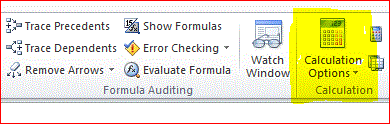## The Issue

Sometimes we are confronted with a worksheet with one or more cells/columns/rows/ranges of cells with  numbers that are formatted as text.  Excel provides an indicator when this is the case, a yellow diamond with an exclamation point.Error Indicator
There are a number of options for resolving this.

## WARNING!!!!!

Converting data can can be a slow process because with each cell's conversion a recalculation is triggered and another piece of information is logged for rollback (undo).  For those of you familiar with the Excel Object model, this is because a conversion of a cell from text to numeric triggers the workbook's WorksheetChange event, which in turn triggers Recalculation.  If the Application.RecalculationMode is set to Automatic, then all of those VLOOKUP()s SUM()s, and other functions are recalculated.

Even if there are no formulas in the workbook, it can take quite a while for the conversion to complete.  As I write this I am converting a full column(1,048,576 cells) of a worksheet (Using the Slow Way).  There are no formulas in the workbook.

I let it run over 30 minutes, sucking up 100% of one of my processors and eating up another 4K of memory every couple of seconds before I killed the process.Calculation Options (Highlighted)

After re-starting Excel, I re-opened the workbook, and reset Calculation Options to Manual.  That is done by clicking the Calculation Options on the Formulas tab of the ribbon then selecting "Manual".

It was done in minutes.

## Make Excel Fix It

### The Tedious Way

The conversions can be done one at a time, and you may wish to do this depending on the circumstances, e.g. only a couple of VLOOKUPs failed with an #N/A error.

The advantage of this method is Excel finds where the numbers are stored as text.
1. Go to the Formulas tab of the ribbon
Excel will now walk you through, row by row beginning with the current selection, all of the cells with an error, including those that have numbers stored as text.

One issue is that since it runs row-by-row.  Trying to focus it by selecting a range before clicking the Error Checking option (as can be done with a Find)  has no effect.

### The Faster Way

Find a corner (top of a column; left-most of a row, upper-left of a rectangle of cells) then click on the down-arrow of the Error Indicator, and select Convert to Number

## Use a Formula

This is the method to use if you still want to maintain the text values for some reason, perhaps there are leading zeros that are important

In another column, typically an adjoining column/row that you may have inserted, use the VALUE() function, then copy it down/across.  Assuming the data is in column A, starting in row 2, and you're putting the VALUE() in column B, in B2 enter (without the quotes) "=VALUE(A2)", then copy it down the column.

## Use a Macro

When using a macro, the Calculation mode can be set to manual then reset to what it was before the macro was run.

Sub ConvertToNumeric()
Dim cel As Range
Dim RecalcState As XlCalculationState
RecalcState = Application.Calculation
Application.Calculation = xlManual
For Each cel In Selection.Cells
If IsNumeric(cel.Value) And WorksheetFunction.IsText(cel.Value) Then
cel.Value = Val(cel.Value)
End If
Next cel
Application.Calculation = RecalcState
End Sub